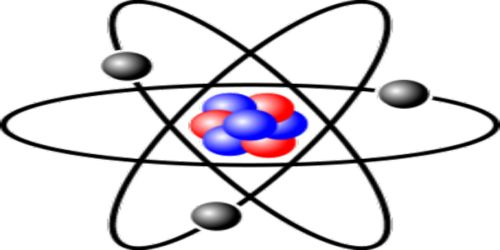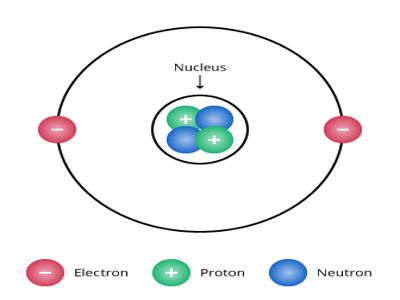Physics

# Proton-neutron TheoryProton-neutron Theory

In 1932, scientist Chadwick discovered neutron. Neutron is a chargeless particle whose mass is about the same mass of the proton. After the discovery of the neutron, scientists came to the conclusion that other than hydrogen all other nucleus consists of proton and neutron. This is the proton-neutron theory.

Let consider a helium nucleus. In its nucleus, there are two protons and two neutrons. Two positive charges of the proton are neutralized by the negative charge of electrons outside the nucleus; as a result, the atom becomes electrically neutral. According to this theory, the structure of the helium nucleus is shown in Figure.Fig.: Formation of He-nucleus according to proton, neutron

A nucleus is expressed by two numbers. One is mass number A and the other one is atomic number Z. About the structure of the atom, scientists have the opinion that other than mass number A and atomic number Z, there are (A – Z) = N number of neutrons and outside the nucleus, there is Z number of electrons. For example, a helium nucleus is designated as 2He4. Here, A = 4, Z = 2. So, in its nucleus, there are two protons and (4 – 2) = 2 neutrons and outside the nucleus, there are two electrons.

In natural radioactivity, following explanations for the emission of beta particle i.e., electron and emission of a position (i.e., positively charged electron or anti-electron) can be given. Actually, electrons do not stay inside the nucleus but during the transformation of the neutron into proton electrons are emitted.

n → p+ + e.

Again, if the proton is transformed into the neutron, the position is emitted.

p+ → n + e+

By the help of proton-neutron theory for the structure of the nucleus, many complicated phenomena of atom and nucleus can be explained.